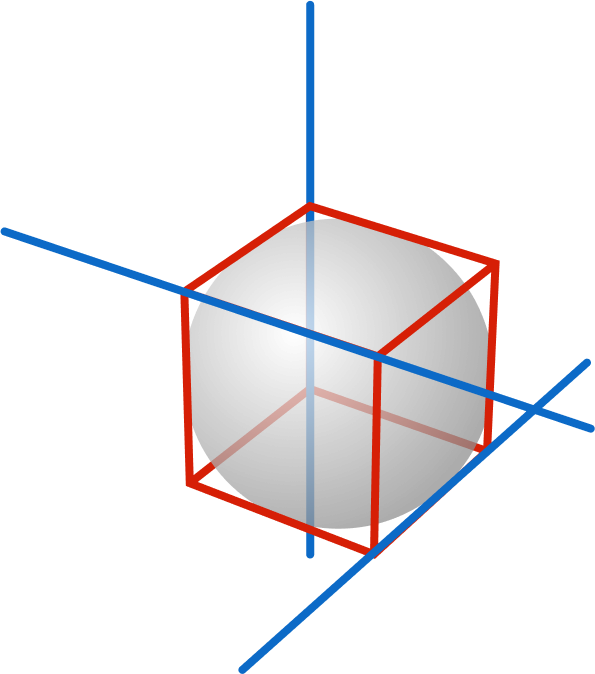# Five and Only Five Spheres

Geometry Level 3Given a unit cube, extend 3 of its edges into non-intersecting, non-parallel lines (in blue) in 3D space. Then five and only five spheres of the same radius are each tangent to all 3 of the lines.

What is the radius of each of these spheres?

The figure at right illustrates a sphere tangent to the 3 blue lines, but there can only be one such sphere with that radius. (Remember, we need five identical spheres satisfying the tangency condition!)

Suppose the radius of each of the five spheres can be expressed as $\frac{a}{b}\sqrt{c},$ where integers $a$ and $b$ are coprime and integer $c$ is square-free.

What is the product $a \times b \times c?$

Note: Spheres may intersect one another.

×